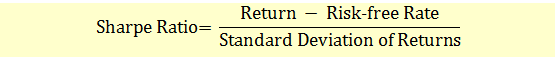# Sharpe Ratio

While deciding about what investments to make, one should weigh the rewards versus the risks of the investment opportunity. The Sharpe ratio is one popular measure of return on risk. It is named after Nobel Laureate professor William F. Sharpe.

The Sharpe ratio measures the reward (or excess return) of an asset per unit of risk.

The Sharpe ratio is also commonly expressed as:Both the return and the standard deviation are annualized. To annualize returns, you multiply linearly by time. For example, a monthly return of 1% converts to an annualized return of 12%. Standard deviation of return is a measure of risk, or uncertainty, of returns. To annualize standard deviation, multiply by the square root of time.

For example, a monthly standard deviation of return of 1 % converts to annualized standard deviation of 1 % x SQRT(12) = 3.46%.

A higher Sharpe ratio indicates better portfolio performance. Sharpe ratios can be increased either by increasing returns or by decreasing risk.

As we know, a portfolio can achieve higher returns by taking on additional risks. Using the Sharpe Ratio one can determine the source of higher returns: better performance or from additional risks.

Historically, Sharpe ratios over long periods of time for most major asset classes have ranged from 0.3 to 2.

Sharpe ratio has two limitations.

1. When the Sharpe ratio is positive, if we increase the risk, the ratio decreases. When Sharpe ratio is negative, however, increasing the risk brings the Sharpe ratio closer to zero, i.e., a higher Sharpe ratio. However, it doesn’t mean better risk-adjusted performance.
2. It considers only the standard deviation as a measure of risk. If the portfolio returns are asymmetric such as strategies involving options, standard deviation is not a good risk measure. For such a portfolio using standard deviation will overestimate Sharpe ratio.